# MCAT Physical : Thermodynamic Systems and Calorimetry

## Example Questions

2 Next →

### Example Question #51 : Physical Chemistry

A metal object that is dropped into water. In which scenario would heat be transferred from the object to the water?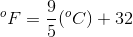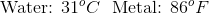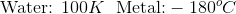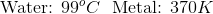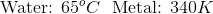Explanation:

If heat is transferred from the metal object to the water, that means that the temperature of the metal must be greater than that of the water. We must find the answer choice where this is the case. Since the temperatures are given in different units, they must be converted to the same unit for comparison purposes.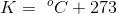Only one answer option results in the metal having a greater temperature than the water: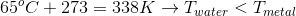### Example Question #51 : Physical Chemistry

A 35g piece of aluminum at a temperature of 373K is placed into 150g of water at a temperature of 298K. The aluminum and water eventually become the same temperature. No heat is released to the surroundings.

Water has a specific heat capacity of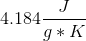and aluminum has a specific heat capacity of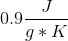.

What is the final temperature of the water and aluminum in the container?

315.5K

324.5K

301.6K

308.9K

301.6K

Explanation:

In this problem, we need to track the transfer of heat from the aluminum to the water. Since the heat acquired by the water is equal to the heat given off by the aluminum, we can set their equations equal to each other; however, in order to avoid a negative number, aluminum's change in temperature will be set as the initial temperature minus the final temperature. This results in the equation below.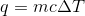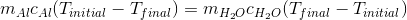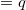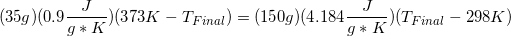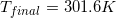### Example Question #1061 : Mcat Physical Sciences

A 200g sample of gold is subjected to 1.2kJ of heat.

The specific heat capacity for gold is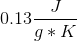.

What is the change in temperature as a result of the heating?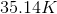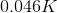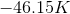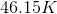Explanation:

When a specific heat capacity is given, we typically use the equation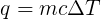. Since we know all of the factors except for the change in temperature, we can simply solve for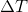.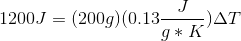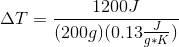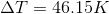### Example Question #3 : Physical Chemistry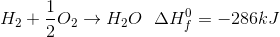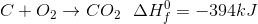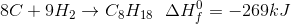Given the enthalpies of formation, what is the enthalpy of combustion of octane in the reaction: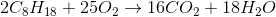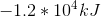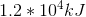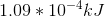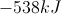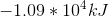Explanation:

The equation for enthalpy of reaction is: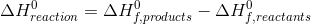Given our chemical reaction and the enthalpies of formation, we can find the enthalpy of reaction.First, find the total enthalpy for the products.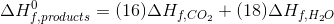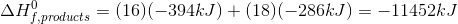Then, find the total enthalpy for the reactants.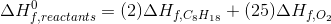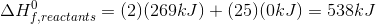Since the oxygen is elemental, its heat of formation is zero.

Return to the original equation to calculate the final enthalpy of reaction.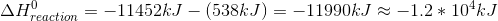2 Next →

### All MCAT Physical Resources